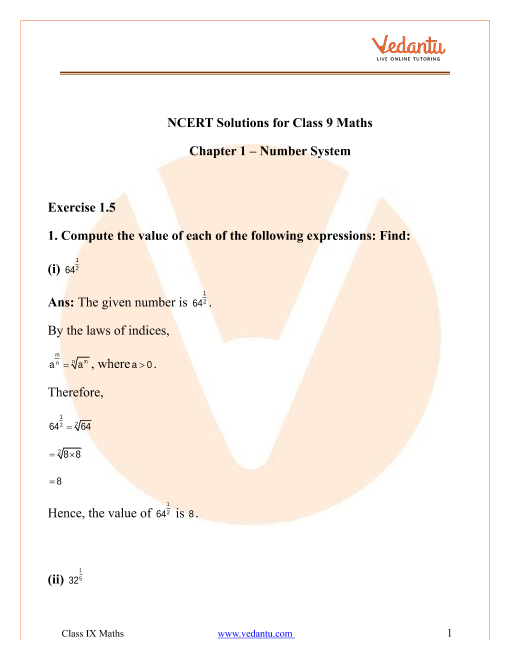# NCERT Solutions for Class 9 Maths Chapter 1 Number Systems (Ex 1.5) Exercise 1.5

## NCERT Solutions for Class 9 Maths Chapter 1 Exercise 1.5 (Ex 1.5)

NCERT Solutions for Class 9 Maths Chapter 1 are about the numbers. There are various types of numbers which have different properties. In this chapter, students will understand how to solve questions on various types of numbers. In Class 9 Maths Chapter 1 Exercise 1.5, you will face problems related to rational and irrational numbers. We, at Vedantu, give you a complete solution to Class 9 Maths Chapter 1 Exercise 1.5. These can be downloaded for free in PDF format.